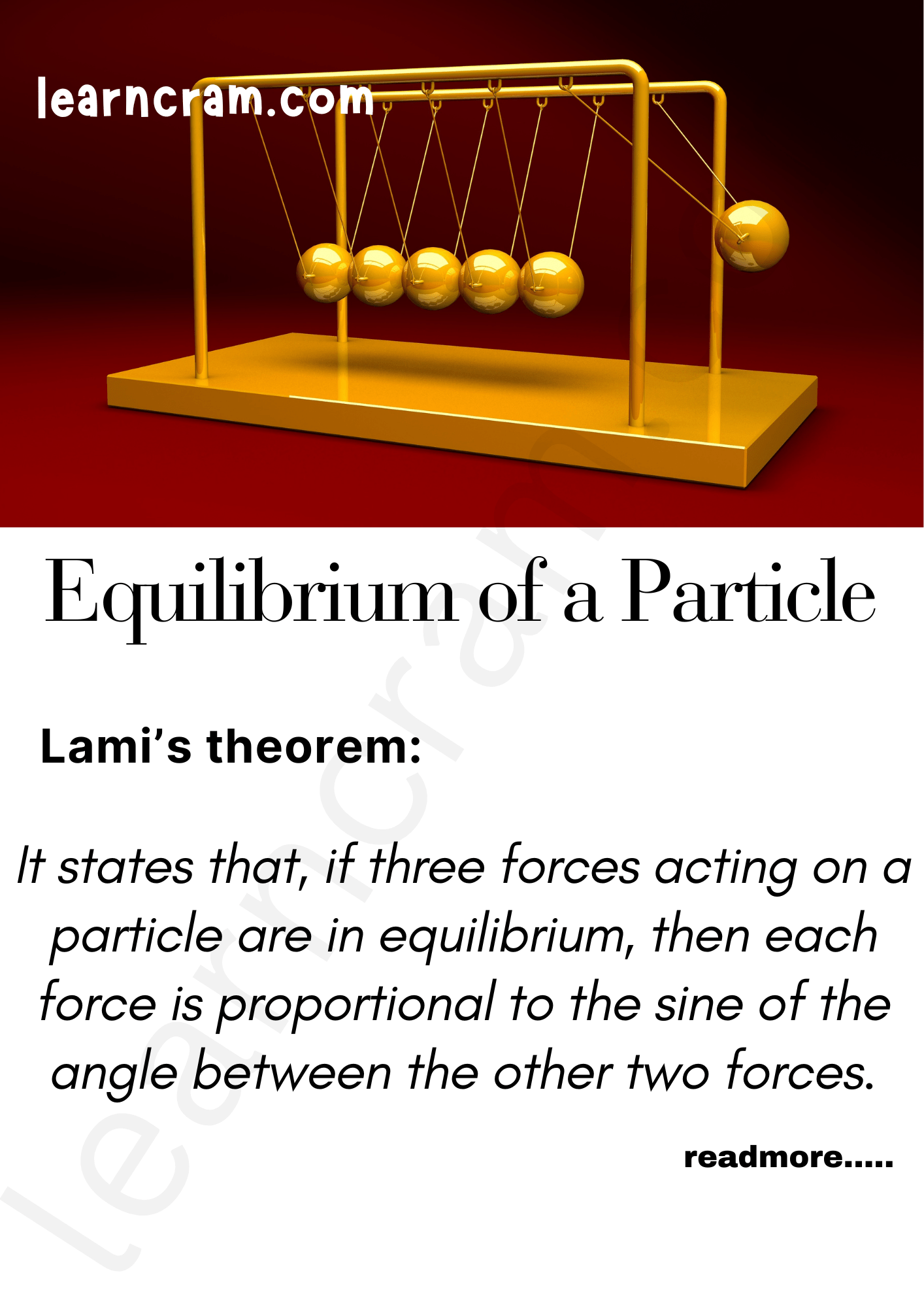# Equilibrium of a Particle Physics | Definition – Laws of Motion

## Equilibrium of a Particle Physics | Definition – Laws of Motion

We are giving a detailed and clear sheet on all Physics Notes that are very useful to understand the Basic Physics Concepts.

Equilibrium of a Particle in Physics:
A particle is in equilibrium if the vector sum of the external forces acting on it is zero.
When the vector sum of the forces acting on a body is zero, then the body is said to be in equilibrium.If two forces F1 and F2 act on a particle, then they will be in equilibrium if F1 + F2 = 0.Lami’s theorem:
It states that, if three forces acting on a particle are in equilibrium, then each force is proportional to the sine of the angle between the other two forces.$$3 \frac{F_{1}}{\sin \alpha}=\frac{F_{2}}{\sin \beta}=\frac{F_{3}}{\sin \gamma}$$Laws of Motion:
There are various laws in Physics that define the motion of the object. When an object is in motion whether it is linear or circular there is some force which is always imposed on it.